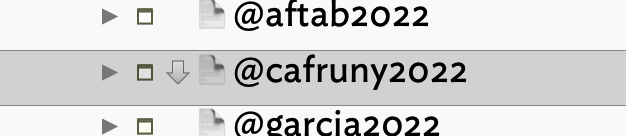# An issue with \$DisplayExpression

Hi!
Here is my \$Edict action code:

``````if(\$ToRead=="true")
{\$DisplayExpression=\$Name + " 📕" + " [" + \$ChildCount + "]"};
if(\$Processing=="true";)
{\$DisplayExpression=\$Name + " 📙" + " [" + \$ChildCount + "]"};
if(\$Done=="true";)
{\$DisplayExpression=\$Name + " 📗" + " [" + \$ChildCount + "]"};
``````

Action code seems to work because I can see the correct Display Expression in the Text Inspector.

But! I can’t see the result in the outline view:Update: It seems to partially work when the note doesn’t have children, but then it just shows \$Name +oror.

Any suggestions?
Thanks!

\$DisplayExpression is an expression — a formula to be evaluated. For example, a \$DisplayExpression might be:

``````\$Name + " 📕" + " [" + \$ChildCount + "]"
``````

Your edict, instead, gets the value of \$Name when the edict runs, appends a symbol, and then appends the child count.

``````Hubert  📕  [ 0 ]
``````

This isn’t a value we can evaluate! So, Tinderbox bails out. Sometimes, it might be close enough to a valid expression that Tinderbox will return something, which is what you’re sometimes seeing.

The best way to set different display expressions in an edict is this:

1. Set up each DisplayExpression in a sample note, perhaps inside the Hints container.

2. In your edict, set the DisplayExpression of this note to the appropriate sample note’s DisplayExpression:

``````if(\$ToRead=="true")